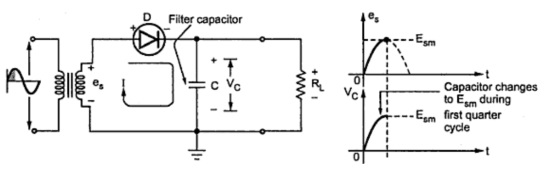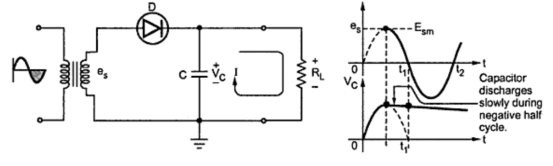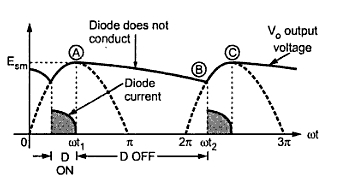### Capacitor Input Filter : Part1

The block schematic of capacitor input filter is shown in the Fig. 1. Looking from the rectifier side the first element in filter is a capacitor.Fig. 1

1.1 Operation of the Filter with Half Wave Rectifier
The Fig. 2 shows a half wave rectifier with a capacitor input filter. The filter uses a single capacitor connected in parallel with the load, represented by the resistance. In order to minimize the ripple in the output, the capacitor C used in the filter circuit is quite large, of the order of tens of microfarads. The half wave rectifier is considered to explain the principle of operation and then the concept is applied to a full wave rectifier.Fig. 2  Operation of capacitor input filter

During the positive quarter cycle of the input signal es, the diode is forward biased. This charges the capacitor C to peak value of input i.e Esm. Practically the capacitor C charges to ( Esm - 0.7) V, due to diode forward voltage drop. This initial charging happens only once, immediately when the power is turned on.
When the input starts decreasing below its peak value, the capacitor remains charged at  Esm and the ideal diode gets reverse biased. This is because the capacitor voltage which is cathode voltage of diode becomes more positive than anode. So during the entire negative half cycle and some part of the next positive half cycle, capacitor discharges through RL as shown in the Fig. 3.Fig. 3  Capacitor discharge through load resistance

The discharging of capacitor is decided by RL C time constant which is very large and hence capacitor discharges very little from Esm. In the next positive half cycle, when becomes more than capacitor voltage, The diode becomes forward biased and charges the capacitor C back to. This is shown in the Fig. 4.Fig. 4  Capacitor starts charging again

The capacitor starts charging at point B where eexceeds the capacitor voltage which is slightly less than Esm. So from B onwards the capacitor starts charging again and gets charged to. The discharging of the capacitor is from A to B.
The capacitor voltage is same as the output voltage as it is parallel with RL. It can be seen that the diode conducts only from point B till capacitor gets charged back to. Thus diode conducts only for part of the positive half cycle. From point A to B, the diode remains nonconducting and conducts only for the period from B to C. This is shown in the Fig. 5.]Fig. 5  Diode conducts only for part of positive cycle

When the diode is nonconducting the capacitor supplies the load current. As the time required by the capacitor is very small to charge while its discharging time constant is very large, the ripple in the output gets reduced considerably.